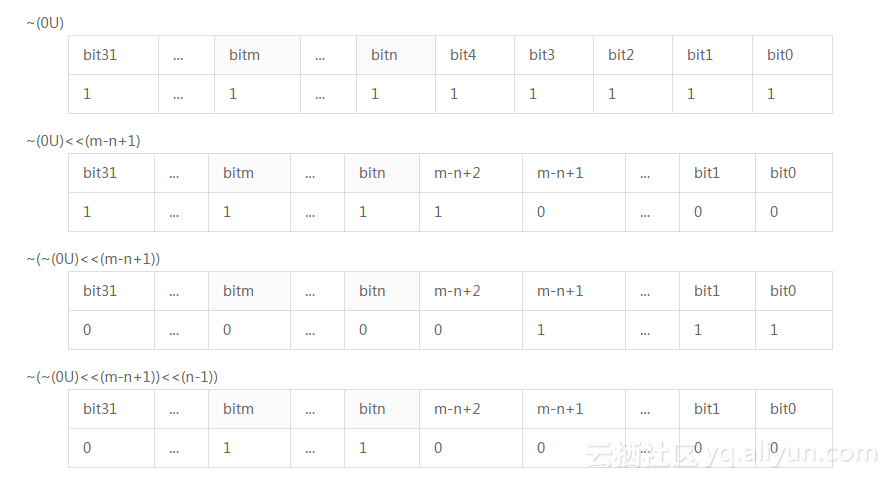+关注继续查看

define GETBITS(x, n, m) ((x & ~(~(0U)<<(m-n+1))<<(n-1)) >> (n-1))Y = (x & ~(~(0U)<<(m-n+1))<<(n-1))

1．嵌入式系统中常常要求用户对变量或者寄存器进行位操作，下面的函数分别用于设置和清除变量a的第5位，请使用下面宏定义bit5，按要求对变量a进行相应的处理，在函数set_bit5中，用位或赋值操作（|=）设置变量a的第5位。在函数clear_bit5中，用位与赋值操作（&=）清除变量a的第5位。（软考题）

define BIT5 (0X01<<5)

static int a;

void set_bit(void)
{

______;

}
void clear_bit5(void)
{

______;

}
2．请描述如下位操作的作用。

a |= (1 << 3);

a |= (0b11111 << 3); 或a |= (~((~0) << 5) << 3);

a &= ~(1 << 15);

a &= ~(0b111111111 << 15); 或a &= ~(~((~0) << 9) << 15);

a &= (0b111111 << 3); 或a &= (~((~0) << 6) << 3);

3．请解释如下两个宏的含义。

#define SET_NTH_BIT(x, n)　(x | ((1U)<<(n-1)))

#define CLEAR_NTH_BIT(x, n) (x & ~((1U)<<(n-1)))

4．截取变量的部分连续位，例如变量0x88, 也就是10001000，若截取第2~4位，则值为010 = 2，最右边从第0位算起，假设m=4，n=2。2956 0FREEBSD上如何使用c语言和libxml2简单解析XML

1612 0Linux下的两个经典宏定义【转】

649 0908 0989 08788 0Android的init过程（二）：初始化语言（init.rc）解析
Android的init过程（一） 本文使用的软件版本 Android：4.2.2 Linux内核：3.1.10     在上一篇文章中介绍了init的初始化第一阶段，也就是处理各种属性。
735 01129 0SAS进阶《深入解析SAS》之开发多语言支持的SAS程序
SAS进阶《深入解析SAS》之开发多语言支持的SAS程序 1. 多语言支持的应用程序是指该程序在世界给第使用时，其能够处理的数据，以及处理数据的方式、信息展现的方式都符合当地的语言、文化习惯，这要求应用程序运行时，能够自动进行与地区、语言相关的处理，也就是通常所说的国际化。 2. SBCS、DBCS、MBCS 单字节字符集SBCS指在该字符集中的字符最多由2个字
1665 0《R语言数据挖掘：实用项目解析》——1.3　数据类型转换
829 0

12049

0

《2021云上架构与运维峰会演讲合集》

《零基础CSS入门教程》

《零基础HTML入门教程》